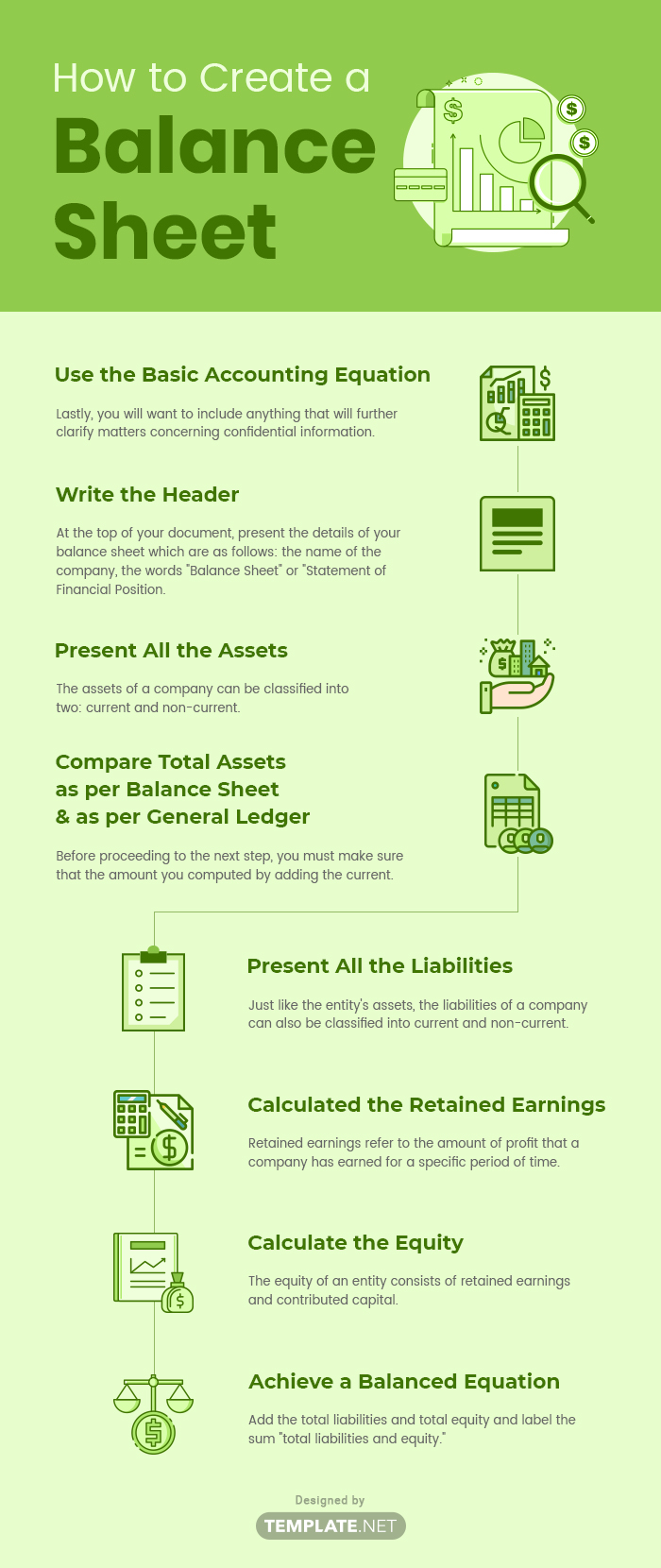## What Is a Balance Sheet?

The balance sheet is one of the components of a financial statement which displays the entity's assets, liabilities, and equity, hence leading to the fundamental equation, assets = liabilities + equity. Also referred to as the statement of net worth or statement of financial position, it is described as the snapshot of the company's financial position at the end of a specified date. It is the basis for computing rates of return and for evaluating the company's capital structure and is key to both financial modeling and accounting.

## How to Create a Balance SheetCreating a balance sheet may overwhelming at first, but when you follow these simple steps, you can surely make an organized and well-structured balance sheet in just a snap.

### 1. Use the Basic Accounting Equation

To start, you must constantly bear in mind the basic equation stated above, which is assets = liabilities + equity. This can also be presented as assets − liabilities = equity. This means that whenever you record a transaction, you must see to it that everything is balanced, such that the amount of the accounts affected in both sides of the equation would equate to zero. Having a deep understanding of this accounting fundamental will surely help you not get easily confused even with complicated transactions.

At the top of your document, present the details of your balance sheet which are as follows: the name of the company, the words "Balance Sheet" or "Statement of Financial Position, and the specific date of the balance sheet which is usually at the end of the calendar year or at the end of the accounting period,.

### 3. Present All the Assets

The assets of a company can be classified into two: current and non-current. Present the current assets first in order of relative liquidity followed by the non-current assets. Current assets are cash, cash equivalents, and those that can be converted into cash within one year of the balance sheet date. Examples of current assets are cash, marketable securities, accounts receivable, inventory, and prepaid expenses. On the other hand, non-current assets are those that can be used for more than one year, for example, property, plant, and equipment. Compute the totals for the current assets and for the non-current assets, and then add up the totals and label this amount "total assets."

### 4. Compare Total Assets as per Balance Sheet and as per General Ledger

Before proceeding to the next step, you must make sure that the amount you computed by adding the current and non-current assets is equal to the amount presented in your general ledger. If you find any differences, investigate and resolve them immediately.

### 5. Present All the Liabilities

Just like the entity's assets, the liabilities of a company can also be classified into current and non-current. Current liabilities are those that are due within one year from the balance sheet date, while non-current liabilities, or long-term liabilities, are those that are otherwise. Examples of current liabilities are accounts payable, accrued liabilities, and short-term notes payable, while examples of non-current liabilities are long-term notes, mortgage payable, and bonds payable. Similar to the assets, compute the total liabilities and label it as "total liabilities."

### 6. Calculated the Retained Earnings

Retained earnings refer to the amount of profit that a company has earned for a specific period of time. In order to compute for the retained earnings, find the ending balance of the retained earnings account from the previous period, add the net income, and deduct the cash dividends paid to investors.

### 7. Calculate the Equity

The equity of an entity consists of retained earnings and contributed capital. The equity accounts are as follows: common stocks, treasury stocks, and retained earnings which you have computed in the previous step.

### 8. Achieve a Balanced Equation

Add the total liabilities and total equity and label the sum "total liabilities and equity." Compare this amount to the total assets, and check if they are balanced. If so, you are now done making your balance sheet. If not, double check your work for you may have omitted or miscategorized an account.